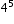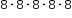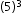# MAFS.6.EE.1.1Archived StandardExport Print
Write and evaluate numerical expressions involving whole-number exponents.
General Information
Subject Area: Mathematics
Domain-Subdomain: Expressions & Equations
Cluster: Level 1: Recall
Cluster: Apply and extend previous understandings of arithmetic to algebraic expressions. (Major Cluster) -

Clusters should not be sorted from Major to Supporting and then taught in that order. To do so would strip the coherence of the mathematical ideas and miss the opportunity to enhance the major work of the grade with the supporting clusters.

Date of Last Rating: 02/14
Status: State Board Approved - Archived
Assessed: Yes
Test Item Specifications

• Assessment Limits :
Whole number bases.

Whole number exponents.

• Calculator :

No

• Context :

Allowable

Sample Test Items (3)
• Test Item #: Sample Item 1
• Question:

Which value is equivalent to the expression?

• Difficulty: N/A
• Type: MC: Multiple Choice

• Test Item #: Sample Item 2
• Question:

Select all the expressions that are equivalent to.

• Difficulty: N/A
• Type: MS: Multiselect

• Test Item #: Sample Item 3
• Question:

What is the value of?

• Difficulty: N/A
• Type: EE: Equation Editor

## Related Courses

This benchmark is part of these courses.
1205010: M/J Grade 6 Mathematics (Specifically in versions: 2014 - 2015, 2015 - 2022, 2022 and beyond (current))
1205020: M/J Accelerated Mathematics Grade 6 (Specifically in versions: 2014 - 2015, 2015 - 2020, 2020 - 2022, 2022 and beyond (current))
1204000: M/J Foundational Skills in Mathematics 6-8 (Specifically in versions: 2014 - 2015, 2015 - 2022, 2022 and beyond (current))
7812015: Access M/J Grade 6 Mathematics (Specifically in versions: 2014 - 2015, 2015 - 2018, 2018 - 2022, 2022 and beyond (current))
7912110: Fundamental Explorations in Mathematics 1 (Specifically in versions: 2013 - 2015, 2015 - 2017 (course terminated))

## Related Access Points

Alternate version of this benchmark for students with significant cognitive disabilities.

## Related Resources

Vetted resources educators can use to teach the concepts and skills in this benchmark.

## Formative Assessments

Cube House:

Students are asked to write a numerical expression using exponents.

Type: Formative Assessment

Exponent Priorities:

Students are asked to evaluate numerical expressions with exponents.

Type: Formative Assessment

Paul’s Pennies:

Students are asked to write and evaluate a numerical expression using exponents.

Type: Formative Assessment

Evaluating Exponents:

Students are asked to expand and evaluate exponential expressions containing whole number exponents.

Type: Formative Assessment

## Lesson Plans

Gather Data For Distribution by Programming an App:

This lesson allow students to gather, calculate, and plot data using both computer code and mathematical equations. In this lesson students will create a pedometer app to demonstrate the understanding of algorithms, components (such as buttons, textboxes, sensors, etc.), and If/Then statements. This lesson uses algebraic equations and random data to access the needed components to store data in a spreadsheet.

Type: Lesson Plan

Data Sets Represented in Computers:

This lesson shows how data can be represented by computers, in relation to everyday activities we may not be aware that we use computer. It gives an overview of graphing data by creating a histogram based on population data. Using the data collected, students will get a chance to hand write code to show what structure is needed for computers to collect, analyze and distribute such data. This lesson is lesson 1 of the Data Set and Deviation Statistics Unit and bridges statistical concepts of data collection, graphing and analysis with programming a computer using coding language while reinforcing foundational algebraic skills.

Type: Lesson Plan

Energy From The Sun To Make Some Fun!:

MSP Racing Company is a cutting edge company that wishes to break through and develop the fastest renewable energy vehicle of its kind. In this lesson, students will be divided into teams of three and will be assigned the task of developing a solar-powered race car to be purchased and developed in full scale by MSP Racing. Each group of students will be employees of MSP Racing Company and will have to develop, test, and present their car to the boss (teacher) of the company.

Type: Lesson Plan

The Power of Exponents :

Students will be introduced to the power of exponents through the parable A Grain of Rice, by Demi. Students analyze the parts of an exponential expression and evaluate the expression using mental math and paper and pencil.

Type: Lesson Plan

The "Power" of Exponents:

The students will use square tiles or cubes to explore the meaning behind the terms "squared" and "cubed" numbers. They will be able to connect understanding between modeling squared and cubed numbers to build a strong foundation for working with exponents. The students also make connections between area and volume models and exponential forms of numbers using whole number exponents. This is an introductory lesson.

Type: Lesson Plan

It's Hip 2b^2 eXponent^s:

Meaning of Exponents... Students will write and simplify numerical and algebraic expressions with whole-number exponents. Bases are limited to positive integers.

Type: Lesson Plan

## Original Student Tutorials

Order of Operations with Fractions:

Evaluate numerical expressions with fractions using the order of operations and properties of operations in this interactive tutorial.

Type: Original Student Tutorial

Order of Operations with Decimals:

Evaluate numerical expressions with decimals using the order of operations and properties of operations in this interactive tutorial.

Type: Original Student Tutorial

Order of Operations with Whole Numbers: Part 2:

Evaluate numerical expressions with whole numbers using the order of operations and properties of operations in this interactive tutorial. This is part 2 of a series on evaluating expressions with whole numbers.

Type: Original Student Tutorial

Order of Operations with Whole Numbers:

Evaluate numerical expressions with whole numbers using the order of operations and properties of operations in this interactive tutorial.

Type: Original Student Tutorial

Order of Operations with Integers:

Evaluate numerical expressions with integers using the order of operations and properties of operations in this interactive tutorial.

Type: Original Student Tutorial

MacCoder’s Farm Part 2: Condition Statements:

Explore computer coding on the farm by using condition and IF statements in this interactive tutorial. You'll also get a chance to apply the order of operations as you using coding to solve problems.

Click below to check out the other tutorials in the series.

Type: Original Student Tutorial

MacCoder’s Farm Part 1: Declare Variables:

Explore computer coding on the farm by declaring and initializing variables in this interactive tutorial. You'll also get a chance to practice your long division skills.

Type: Original Student Tutorial

The Djinni’s Offer:

Students are asked to explore and then write an expression with an exponent. The purpose of this task is to introduce the idea of exponential growth and then connect that growth to expressions involving exponents. It illustrates well how fast exponential expressions grow.

Watch out for Parentheses:

This problem asks the student to evaluate three numerical expressions that contain the same integers yet have differing results due to placement of parentheses.

## Student Center Activity

Students can practice answering mathematics questions on a variety of topics. With an account, students can save their work and send it to their teacher when complete.

Type: Student Center Activity

## Tutorials

Powers of Zero:

Students will learn that non-zero numbers to the zero power equal one. They will also learn that zero to any positive exponent equals zero.

Type: Tutorial

Patterns in Raising 1 and -1 to Different Powers:

You will discover rules to help you determine the sign of an exponential expression with a base of -1.

Type: Tutorial

Introduction to Order of Operations:

Students will evaluate expressions using the order of operations.

Type: Tutorial

Order of Operations :

This video will show how to evaluate expressions with exponents using the order of operations.

Type: Tutorial

Introduction to Exponents:

This video demonstrates how to evaluate expressions with whole number exponents.

Type: Tutorial

The Zero Power:

Learn why a number raised to the zero power equals 1.

Type: Tutorial

## Unit/Lesson Sequence

Using the Laws of Arithmetic:

This lesson unit helps teachers assess how students perform arithmetic operations, write and evaluate numerical expression s from diagrammatic representations, apply the distributive and commutative properties, and find areas of compound rectangles. The time for the lesson is broken into a 15 minute pre-lesson task, an 80 minute lesson (or two 40 minute lessons), and a 15 minute follow up lesson or homework. Individual students will need a copy of the two tasks, a mini-whiteboard, a pen, and an eraser. Each small group will need cut-up copies of the card sets, a large sheet of poster-sized paper, and a glue stick.

Type: Unit/Lesson Sequence

## Virtual Manipulative

Order of Operations Quiz:

In this activity, students practice solving algebraic expressions using order of operations. The applet records their score so the student can track their progress. This activity allows students to practice applying the order of operations when solving problems. This activity includes supplemental materials, including background information about the topics covered, a description of how to use the application, and exploration questions for use with the java applet.

Type: Virtual Manipulative

## MFAS Formative Assessments

Cube House:

Students are asked to write a numerical expression using exponents.

Evaluating Exponents:

Students are asked to expand and evaluate exponential expressions containing whole number exponents.

Exponent Priorities:

Students are asked to evaluate numerical expressions with exponents.

Paul’s Pennies:

Students are asked to write and evaluate a numerical expression using exponents.

## Original Student Tutorials Mathematics - Grades 6-8

Order of Operations with Decimals:

Evaluate numerical expressions with decimals using the order of operations and properties of operations in this interactive tutorial.

Order of Operations with Fractions:

Evaluate numerical expressions with fractions using the order of operations and properties of operations in this interactive tutorial.

Order of Operations with Integers:

Evaluate numerical expressions with integers using the order of operations and properties of operations in this interactive tutorial.

Order of Operations with Whole Numbers:

Evaluate numerical expressions with whole numbers using the order of operations and properties of operations in this interactive tutorial.

Order of Operations with Whole Numbers: Part 2:

Evaluate numerical expressions with whole numbers using the order of operations and properties of operations in this interactive tutorial. This is part 2 of a series on evaluating expressions with whole numbers.

## Computer Science Original Student Tutorials

MacCoder’s Farm Part 1: Declare Variables:

Explore computer coding on the farm by declaring and initializing variables in this interactive tutorial. You'll also get a chance to practice your long division skills.

MacCoder’s Farm Part 2: Condition Statements:

Explore computer coding on the farm by using condition and IF statements in this interactive tutorial. You'll also get a chance to apply the order of operations as you using coding to solve problems.

Click below to check out the other tutorials in the series.

## Student Resources

Vetted resources students can use to learn the concepts and skills in this benchmark.

## Original Student Tutorials

Order of Operations with Fractions:

Evaluate numerical expressions with fractions using the order of operations and properties of operations in this interactive tutorial.

Type: Original Student Tutorial

Order of Operations with Decimals:

Evaluate numerical expressions with decimals using the order of operations and properties of operations in this interactive tutorial.

Type: Original Student Tutorial

Order of Operations with Whole Numbers: Part 2:

Evaluate numerical expressions with whole numbers using the order of operations and properties of operations in this interactive tutorial. This is part 2 of a series on evaluating expressions with whole numbers.

Type: Original Student Tutorial

Order of Operations with Whole Numbers:

Evaluate numerical expressions with whole numbers using the order of operations and properties of operations in this interactive tutorial.

Type: Original Student Tutorial

Order of Operations with Integers:

Evaluate numerical expressions with integers using the order of operations and properties of operations in this interactive tutorial.

Type: Original Student Tutorial

MacCoder’s Farm Part 2: Condition Statements:

Explore computer coding on the farm by using condition and IF statements in this interactive tutorial. You'll also get a chance to apply the order of operations as you using coding to solve problems.

Click below to check out the other tutorials in the series.

Type: Original Student Tutorial

MacCoder’s Farm Part 1: Declare Variables:

Explore computer coding on the farm by declaring and initializing variables in this interactive tutorial. You'll also get a chance to practice your long division skills.

Type: Original Student Tutorial

The Djinni’s Offer:

Students are asked to explore and then write an expression with an exponent. The purpose of this task is to introduce the idea of exponential growth and then connect that growth to expressions involving exponents. It illustrates well how fast exponential expressions grow.

## Student Center Activity

Students can practice answering mathematics questions on a variety of topics. With an account, students can save their work and send it to their teacher when complete.

Type: Student Center Activity

## Tutorials

Powers of Zero:

Students will learn that non-zero numbers to the zero power equal one. They will also learn that zero to any positive exponent equals zero.

Type: Tutorial

Patterns in Raising 1 and -1 to Different Powers:

You will discover rules to help you determine the sign of an exponential expression with a base of -1.

Type: Tutorial

Introduction to Order of Operations:

Students will evaluate expressions using the order of operations.

Type: Tutorial

Order of Operations :

This video will show how to evaluate expressions with exponents using the order of operations.

Type: Tutorial

Introduction to Exponents:

This video demonstrates how to evaluate expressions with whole number exponents.

Type: Tutorial

The Zero Power:

Learn why a number raised to the zero power equals 1.

Type: Tutorial

## Virtual Manipulative

Order of Operations Quiz:

In this activity, students practice solving algebraic expressions using order of operations. The applet records their score so the student can track their progress. This activity allows students to practice applying the order of operations when solving problems. This activity includes supplemental materials, including background information about the topics covered, a description of how to use the application, and exploration questions for use with the java applet.

Type: Virtual Manipulative

## Parent Resources

Vetted resources caregivers can use to help students learn the concepts and skills in this benchmark.